﻿ A Time Series Analysis of Federal Budgetary Allocations to Education Sector in Nigeria (1970-2018)Publications are Open
Access in this journal
Article Versions
Export Article
• Normal Style
• MLA Style
• APA Style
• Chicago Style
Review Article
Open Access Peer-reviewed

### A Time Series Analysis of Federal Budgetary Allocations to Education Sector in Nigeria (1970-2018)

N. M. Eze , O. C. Asogwa, A. O. Obetta, K. C. Ojide, C. I. Okonkwo
American Journal of Applied Mathematics and Statistics. 2020, 8(1), 1-8. DOI: 10.12691/ajams-8-1-1
Received December 02, 2019; Revised January 03, 2020; Accepted January 10, 2020

### Abstract

This work is carried out to statistically analyze federal budgetary allocations to the education sector in Nigeria (1970-2018). Time series analysis is used to analyse the data using the Box and Jenkins modeling approach. This involved identification of model, estimation of parameters, diagnostic checking of the model and forecasting. ARIMA (1, 1, 1) was identified in the course of identification of the model. ARIMA (1, 1, 0) was selected as a parsimonious model using the Bayesian Information Criterion (BIC). Also, the diagnostic check was carried out and it was found that the model is adequate. Forecasts are equally made for the year 2019, 2020, 2021 and 2022 using the model obtained. It was observed that there is an upward trend from 2019-2022 forecasts, hence using the model will bring about increment in the future budgets.

### 1. Introduction

Education is a process of imparting and acquiring of knowledge through teaching and learning especially at a school or similar institution 1. One cannot overemphasize that education plays important role in individual’s and national development. Education, however, needs a lot of funds to achieve its objectives. For examples, money is needed to pay the salaries and allowances of teachers and non-teachers in schools/educational institutions, purchase equipment and facilities needed for the training of students in the institutions, construct blocks of classrooms for the teaching of students and other overhead expenses, just to mention but a few.

Funds for education development can come from various sources, the major source being government, which may be at national, state, or local government level, such fund is at the prerogative of government in power and subject to the amount in coffers of government each year. For this kind of fund to be released to education, there is need to be legislation on it by politicians. In another vein, funds for education can come from non-government sources, such as the private sector, Non-Governmental Organizations (NGOs), and philanthropist. In addition to these, there are the donations from individuals, such as Alumni/old students Association members, and internally generated revenue through business ventures, fund raising involving the schools. In spite of these numerous sources of financing of education in Nigeria, education has not been enjoying commensurate financing as other major sectors in the country. 2, 3, reiterate that challenges facing the management of the college and university system are rooted in inadequate funding. One of the approaches the government adopts in financing education in Nigeria is the annual budgetary provision to the sector which is distributed as subvention/grants to the different levels of the educational system. Over the years, financial allocation to education sector in Nigeria leaves much to be desired. The trends of financial allocation to the sector, right from the pre-independence to the present moment, reveals fluctuating and low allocation. The trends even fell short of the recommendation of the United Nations Education Scientific and Cultural Organization (UNESCO) that the developing countries should allocate at least the 26 percent of their total annual budget to the education sector. 4 indicated that the quality of teaching and research has fallen considerably because of lack adequate teaching and research materials resulting from inadequate financing. Notwithstanding, there are observed problems to funding of education; these include; inconsistent funding and lack of suitable model to make budget provisions.

Therefore, this study ought to look at a statistical analysis of federal budgetary allocations to education sector in Nigeria. This is obtained by employing a type of time series modeling known as ARIMA (Auto Regressive integrated Moving Average) modeling. The box-Jenkins approach is used for testing the adequacy of the model. Time series is the set of observations taken at specific time periods usually at equal intervals. It is a sequence of observations of a random process arranged according to their time of occurrence. Such Observations could be daily or weekly sales of a company, monthly record of rainfall statistics during a rainy season, yearly budgetary allocations by the federal or state government etc. One objective of time series is to make inferences regarding basic features of the stochastic process from the information contained in the historical time series. Mathematically, time series analysis is defined by the values (X1, X2,…, Xn) of the variables X at time (t1,t2,..,tn). Hence, X is a function of time, t which is represented as X = f(t).

A number of scholars and researchers have conducted studies in analyzing federal government budgetary allocations to education sector in Nigeria. As cited in 5, Nigerian government over the years has not met the UNESCO recommendation of 26% of the total budget allocation to the education sector. It was revealed that annual budget allocation to education has not gone beyond 8.5% in contrast to the 26% recommended by UNESCO. In fact the allocation has been dwindling.

6 used Ordinary Least Squares method to study fifty transitional and developing countries. It was shown from their results that there is a positive impact on primary and secondary education through high public expenditure on education. Other factors like sanitation, urbanization, and per capita income were also noticed to be help tools to affect enrolment rates and performance of the education sector.

7 studied the determinants of federal government expenditure on education sector in Nigeria using the Ordinary Least Squares approach. The study showed that the expenditure on education sector in Nigeria has been unstable and this is caused by instability in sources of government revenue. The work recommended a development of multiple sources of government revenue and this helps in making consistent funding of the education sector

8 asserted that funding of education constitutes an aspect of public finance due to the fact that the federal, state and local governments are involved in its provision and management. It constitutes a vital aspect of private investment because voluntary organizations and individuals are involved in it.

9 studied the effect of education investment on economic growth in Nigeria through the use of Johansen’s co-integration approach. From the result, it was noticed that government spending on education and expected economic growth have a long-run relationship between them.

10 studied on the impact of state-specific spending on social sectors with main emphasis on education in the 36 states across Nigeria by using fixed and random effect model. It was noticed that there was a significant impact on primary, secondary and adult education enrolments in the states.

11 used Ordinary Least Squares method to assess the effect of capital and recurrent expenditure on education growth in Nigeria. It was shown from the result that the expenditure of government on education is low and this causes lack of education improvement in the country.

### 2. Materials and Methods

The data used in this research work are secondary data. They were sourced from www.cbn.govstatbulletin 12. The data are on the federal budgetary allocations to education sector in Nigeria. They were obtained on annual bases as given in the CBN’s (Central Bank of Nigeria) statistical bulletin 2018. It spans from 1970-2018 based on the fiscal years. We ought to fit an appropriate time series model to these data using ARIMA model. This model will be used to make description, explanation, prediction and control (if necessary). It should be noted that data used for this work only reflect the funds appropriated to this sector within the stipulated years. In other words, the amount that was actually put in use is not captured in this research study.

In this work, an approach given by 13 known as Box and Jenkins approach would be fully employed for the analysis of the obtained data. The Box - Jenkins Analysis refers to a systematic method of identifying, fitting, checking, and using integrated autoregressive moving average (ARIMA) time series models 14.

The Box-Jenkins method refers to the iterative application of the following three steps:

i). Identification. The model identification will be done using plots of the data, autocorrelations, partial autocorrelation. Through these models identifications method, a class of simple ARIMA model is selected. This amounts to estimating appropriate values for p, d, and q.

ii). Estimation. The phi’s and thetas of the selected model are estimated using maximum likelihood techniques, Yule walker equation and Least Square technique.

iii). Diagnostic Checking. The adequacy of the fitted model is tested by considering the autocorrelations and partial autocorrelations of the residual series.

• Figure 1. Graph of budgetary allocations against the years
2.1. Methodology

Usually the first step in analyzing a time series data is to plot the observations against the different time periods. This is known as time plot. Here, we plot the data on federal allocations to education sector against the corresponding years. This is to ascertain the presence of such features as seasonality, trend, cycles, discontinuities and outliers. Graph of the data can also be used for stationarity check.

From Figure 1, it can be observed that the data are not stationary following the presence of a possible upward trend. Moreover, the data are not seasonal since the data are on annual basis.

Test for Stationarity Using ADF (Augmented Dickey Fuller) Test

This is a test to examine the stationarity of data. This test statistic was propounded by Dickey and Fuller in 1979 15

Test Statistic: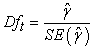Hypotheses: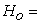There is a unit root (data are not stationary)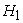= Data are stationary

α= 0.05

Decision Rule: Reject the null hypothesis if the p-value < α-value; otherwise do not rejectConclusion: From Table 1; since p-value = 0.2631 is not less than α=0.05; we do not reject the null hypothesis and therefore conclude that the federal budgetary allocations are not stationary at α= 0.05.

The plot in Figure 2 shows that the differenced data on federal allocations are stationary. This is because the plot shows a constant mean, variance and auto covariance.

Test for Normality

The normality test is carried out to find out whether the data are normally distributed. The one sample kolmogorov-smirnov test is applied as follows

H0: The data follow a normal distribution

H1: The data do not follow a normal distribution

α=0.05(where α is the level of significance)

Decision Rule: Reject the null hypothesis if the p-value < 0.05; otherwise do not reject.

Conclusion: From Table 2; since the p-value = 0.001 is less than α=0.05, we reject the null hypothesis, H0 and therefore, conclude that the data do not follow a normal distribution at α=0.05.

Having observed that the data are not normally distributed, it suffices to obtain the transformation of the data using the method of log transformation. However, conducting a one sample kolmogorov-smirnov test using the transformed data shows that the log transformed data are normally distributed.

Hypotheses:

H0: The data are normally distributed

H1: The data are not normally distributed

α=0.05(where α is the level of significance)

Decision Rule: Reject the null Hypothesis if the p-value < α =0.05; otherwise do not reject the null hypothesis.

Conclusion: Since the p-value =0.200 is not less than α =0.05, we do not reject the null hypothesis and therefore, conclude that the data ate normally distributed at α =0.05.

Also, a look at the Q-Q plot of the transformed data showed that the log transformed data are normally distributed.

• Figure 2. Graph of differenced federal budgetary allocations to education against the years
• Figure 3. The quantile-quantile (q-q) plot

The data are close to the ideal normal distribution looking at the points on the line of the Q-Q plot. This indicates that the data are normally distributed

2.1.1. Autoregressive Process

The autoregressive process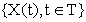is a stochastic model that is very useful in the model presentation of certain real life occurrences that occur in series. In the autoregressive model, current value of the process is expressed as a finite linear aggregate of previous value of the process 16. The model is represented as follows: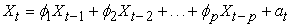(1)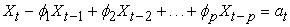(2)(3)

Where;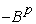is known as the characteristic polynomial of order p;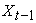is the previous value of the series.

This model can be written as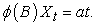2.1.2. The Moving Average Process

In time series analysis, the moving average model (MA model) also known as moving average process, is a common approach for the modeling univariate time series. The moving average model specifies that the output depends linearly on the current and various past values of a stochastic (imperfectly predictable) term. Together with the autoregressive (AR) model, the moving- average model is a special case and key component of the more general ARMA 17 and ARIMA models of time series, which have a more complicated stochastic structure.

Contrary to the AR model, the finite MA model is always stationary. It is represented as: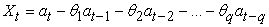(4)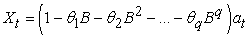(5)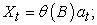where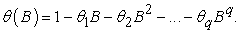And is known as the characteristic polynomial of MA of order q. This process is thought of as the outputfrom a linear filter with transfer function θ(B) which the output is a white noise,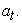2.1.3. Autoregressive Integrated Moving Average (ARIMA) Process

The ARIMA (p,d,q) modeling is a time series modeling approach employed when a time series data are not stationary and needs to be differenced 18. Mathematically, a time seriesis said to follow an integrated Autoregressive Moving Average model if the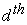difference,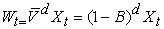is a stationary ARMA process. If we follow an ARMA (p,q) process we say thatis an ARIMA(p,d,q) process.

Where;

P= order of autoregressive model

D=order of differencing

Q=order of moving average process

Data Analysis: Using the Box-Jenkins modeling approach explained above, the three main steps involved in the process are as follows:

1. Model identification

2. Model fitting

3. Model diagnostic

2.1.4. Model Identification

As explained above, the model would be identified using the Autocorrelation function (ACF) and Partial Autocorrelation function (PACF). The behaviours of the Autocorrelation and Partial Autocorrelation are examined carefully. Recall, that for identification to take place the series has to be stationary. The theoretical pattern of ACF and PACF is shown below.

Table 6 shows how to identify models of Autoregressive, Moving Average and Autoregressive Moving average processes using Autocorrelation and Partial autocorrelations.

• Figure 4. Plot of Autocorrelation Function
• Figure 5. Plot of Partial Autocorrelation Function (PACF)

Having obtained the plots of ACF and PACF from the stationarized data, we therefore observe the two plots shown in Figure 4 and Figure 5 above to identify the proper time series model.

The ACF plot shown in Figure 4 above shows exactly the same feature as that of the PACF plot given in Figure 5, both cutting off at lag 1. This however, suggests an ARIMA (1, 1, 1) process model given as;(7)

Having identified the models above, we now compare the following models in the table below to pick the model with the least Bayesian information criterion (BIC), least Mean Absolute Error (MAE) and maximum R-squared.

Therefore, from Table 5, it is clearly shown that the model ARIMA (1,1, 0), has the minimum BIC, minimum MAE and Maximum R-squared therefore we adopt the equation (8) below as the suitable model;(8)

2.1.5. Estimation of Parameters in the Model

Having identified the time series (federal allocations to education) model by studying the ACF and PACF of the time series data and also studying model summary table above, it now suffices to estimate the parameter(s) of the identified model 19. Hence from equation (8) above i.e.,we estimate the parameter, ɸ. The parameter, ɸ can be estimated in the following ways:

1. Least square method

2. Yule walker equation method of estimation

3. An approximation to the maximum likelihood estimation.

1. The Least square estimates for AR(1) process are given as:(9)

and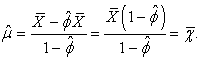(10)

Therefore, from Table 6, the model is fitted as follows

From equation (8) above i.e.,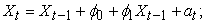Where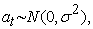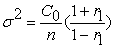but;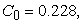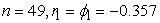(where,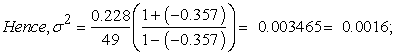But,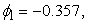= -0.821 (not significant)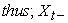=Meanwhile, Stationarity condition for AR(1) process is given as (-1< ɸ1<1); this implies that ɸ1 must lie within +1 and -1.

Since, ɸ is less than 1 (i.e ɸ1= -0.357), then the process is stationary.

2.1.6. Diagnostic Checking of the ARIMA (1,1,0) Model

Having estimated the parameters of the model, we now test the adequacy of the model obtained from the series. If the model is adequate, then the residuals should behave as a white noise (random). We then use the Ljung- Box Q statistics, ACF and PACF of the residuals to check for the adequacy of the model 20, 21.

The Ljung –Box Q-statistic is defined as;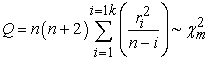Where;

n= number of observations in the series

k= the largest lag( i.e from i=1,2,3,4,…,16)= the sample autocorrelation function at lag i of the residual series

Q has approximately the chi-squared distribution with (k-p-d-q) degree of freedom for ARIMA(p,d,q). Q is the Ljung-Box test statistic for checking the adequacy of the model.

m = the (k-p-d-q) degree of freedom of the chi squared distribution

Hypothesis Testing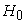= The model is adequate= The model is not adequate

α = 0.05

Test statistic:

Q-statistic=n (n+2)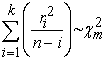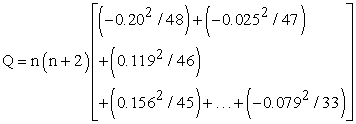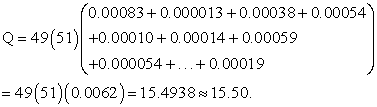Decision Rule: Rejectifotherwise do not rejectConclusion: Since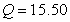is not greater than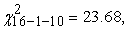we do not rejectand therefore conclude that the model is adequate at α=0 .05.

• Figure 6. Plot of Residuals of ACF and PACF

Careful observation of Figure 6 shows they fall within a 95% confidence interval. This very well shows that the residual process is a white noise. Moreover, it shows that the model is adequate and can be used to make forecast.

### 3. Forecasting

An important use of time series model is to provide forecasts and sometimes the performance of a time series model and indeed of any other model is judged on the basis of this forecasting performance 22, 23, 24. For AR (1) process, the forecast is given by: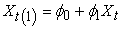(11)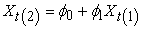(12)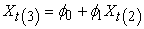(13)

: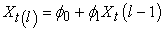(14)

for L>1.

Therefore, from the ARIMA (1,1,0) obtained , the forecasts are given in the table below

Using the model we obtained, the forecast for 2019 and 2020 as 9.83 and 9.87 respectively (see Table 9 above); this translates to N6,760,829,754 (N6.7B) and N7,413,102,413 (N7.4B) for 2019 and 2020 respectively. Hence, using the model to forecast future budgets for the sector shows an increase in the budgetary allocations, hence the model is suitable for making predictions.

• Figure 7. Plot of the Forecast

A look at Figure 7 shows that there is an upward trend from the 2019-2022.

Therefore, this shows that using the model we can obtain the required forecast of the budgets for the sector.

### 4. Summary

In summary, the researchers have been able to fit a model to the data on federal budgetary allocations to education sector from 1970-2018 (www.cbn.govstatbulletin). It was observed from the Autocorrelation function (ACF) and the partial Autocorrelation Function (PACF) plots that the data were not stationary. First order differencing was carried out to make the data stationary. ARIMA (1,1, 1) model was identified from careful observation of the ACF and PACF plots of the differenced data. Both of the plots cutting off after lag 1. Furthermore, BIC (Bayesian information criterion) was used in model selection. Also, Ljung-box test was used in testing whether the model is adequate and it was found that the model is adequate. This means that the residuals have been transformed into a white noise hence, the model can be used to make forecast.

4.1. Conclusion

In conclusion, it was shown that the model is adequate, therefore we can use the model to make future prediction(s) of budgetary allocations to the sector. Therefore, the model can be used as a working tool in drafting future budgets for the sector. Meanwhile, Using the model we obtained the forecast for 2019 and 2020 as 9.83 and 9.87 respectively (see Table 9 above); this translates to N6,760,829,754 (N6.7B) and N7,413,102,413 (N7.4B) for 2019 and 2020 respectively. Hence, this shows a suitable model for drafting future budgets for the sector.

4.2. Recommendation

In order to avoid the problems earlier mentioned in this study which include; inconsistent funding and lack of suitable model with which to draw adequate budgetary provisions for the sector, the researcher recommends that the federal Ministry of Education should use the identified model in its budgetary provisions to education sector. This will solve the problem of inconsistent funding.

### Acknowledgements

Central Bank of Nigeria was strongly acknowledged for making the data for this research available.

### References

Published with license by Science and Education Publishing, Copyright © 2020 N. M. Eze, O. C. Asogwa, A. O. Obetta, K. C. Ojide and C. I. OkonkwoThis work is licensed under a Creative Commons Attribution 4.0 International License. To view a copy of this license, visit http://creativecommons.org/licenses/by/4.0/